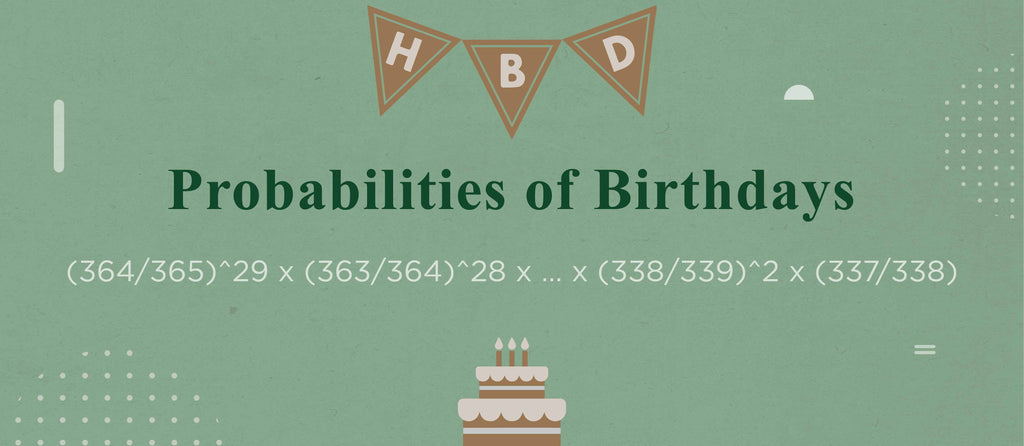Probabilities of Birthdays

Birthdays are like buses. You wait for months for a mate to throw a celebration and then suddenly you have two on one day! As well as an inconvenience for your schedule, it will also throw off your budget for buying birthday presents! But what is the mathematics behind the distribution of birthdays and is there any formula that can assist your planning for gift giving?

Going back to school (in more than one sense): if you are one of a class of 30 pupils, the chance someone else shares their birthday with yours is relatively low. Ignoring leap years for simplicity, there are 365 possibilities for the birthdays of each person. 364 of these will not coincide with yours and so the probability that a specific individual does not share your birthday is 364/365. If we also assume that the distribution of the other 29 students in the class are independent of each other (there are no twins for example) then the chance that each of those students does not share your birthday would be calculated by (364/365)^29 = 0.923521. Very likely, but there is still just under an 8% chance that you’ll be sharing the spotlight.

If instead we consider the problem of any two students sharing a birthday the question changes considerably. One way to approach the problem is to use the solution you have just calculated for your own birthday and then work your way through each student focusing on a specific birthday another 29 times. In that case your calculation will look something like (364/365)^29 x (363/364)^28 x … x (338/339)^2 x (337/338).  Effectively this is multiplying the probability no one shares a birthday with you, by the chance that ‘student A’ doesn’t share a birthday with the remaining 28 students (as you already know they don’t share with you) and so on until only two students remain.

This is a simple method but quite time consuming if you don’t have a computer to run it for you. Approaching the problem slightly differently it’s possible to simplify it. As previously stated, the probability ‘Student A’ does not share a birthday with you is 364/365. There are 363 possibilities for the birthday of ‘Student B’ that will not coincide with either  yours, or ‘Student A’’s birthday and so the probability of this is 363/365. Continuing this for each student, the probability that the final student in the class does not share a birthday with any of the 29 others is 365-29/365. Combining the probabilities of each of these independent events will be 364/365 x 363/365 x …. 336/365 which is equivalent to

And so somewhat surprisingly the chance that at least two students share a birthday is about 70%, or in other words, according to maths, you’ll likely have a few presents to buy.

Unfortunately knowing these probabilities will in no way help you in deciding what gift to give… If you’ve made it this far through these calculations however, a maths equation mug or tesla themed tee could well be something your pals will appreciate.  Check out https://beautifulequations.co.uk/ for more science apparel and gifts!